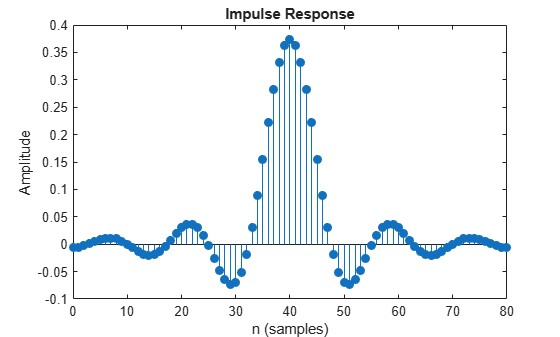# impz

Impulse response of discrete-time filter

## Syntax

``````[impresp,t] = impz(rcfilter)``````
``````[impresp,t] = impz(rcfilter,n)``````
``````[impresp,t] = impz(rcfilter,n,fs)``````
``````[impresp,t] = impz(rcfilter,[],fs)``````
``````[impresp,t] = impz(___,'Arithmetic',arithType)``````
``impz(rcfilter)``

## Description

example

``````[impresp,t] = impz(rcfilter)``` returns `impresp`, the impulse response of the specified filter. The output `t` represents the sample intervals and equals [0 1 2 ...k-1]'. k is the number of filter coefficients.```
``````[impresp,t] = impz(rcfilter,n)``` computes the impulse response at `floor`(`n`) 1 second intervals. In this case, `t` equals (0:`floor`(`n`) – 1)'.```
``````[impresp,t] = impz(rcfilter,n,fs)``` computes the impulse response at `floor`(`n`) 1/`fs` second intervals. In this case, `t` equals (0:`floor`(`n`) – 1)'/`fs`.```
``````[impresp,t] = impz(rcfilter,[],fs)``` computes the impulse response at k 1/`fs` second intervals. k is the number of filter coefficients. In this case, `t` equals (0:k – 1)'/`fs`.```
``````[impresp,t] = impz(___,'Arithmetic',arithType)``` specifies the type of arithmetic that the function uses to evaluate the impulse response. You can use any input combination from the previous syntaxes.```
````impz(rcfilter)` plots the magnitude and unwrapped phase of the impulse response of the specified filter by using the `fvtool` object function.You can use the `impz` object function for real and complex filters. When you omit the output arguments, `impz` plots only the real part of the impulse response.For more input options, see the Signal Processing Toolbox™ `impz` function.```

## Examples

collapse all

Evaluate the impulse response of an RRC filter.

Create a transmit RRC filter System object™. Evaluate the impulse response by using the `impz` object function.

```rcfilter = comm.RaisedCosineTransmitFilter; impz(rcfilter)```## Input Arguments

collapse all

Filter, specified as one of these System objects.

Number of filter coefficients, specified as a positive integer. This value determines the length of the output impulse response vector, `impResp`.

Data Types: `double`

Sampling frequency that the function uses to compute the impulse response, specified as a positive scalar.

Data Types: `double`

Arithmetic used in the filter analysis, specified as `'double'`, `'single'`, or `'Fixed'`. When you do not specify the arithmetic type and the filter System object is unlocked, the analysis tool assumes a double-precision filter. When you do not specify the arithmetic type and the System object is locked, the function performs the analysis based on the data type of the locked input.

The `'Fixed'` value applies to filter System objects with fixed-point properties only.

When you specify this input as `'Fixed'` and the filter object has the data type of the coefficients set to `'Same word length as input'`, the arithmetic analysis performed depends on whether the System object is unlocked or locked.

• If the System object is unlocked, the analysis object function cannot determine the data type of the coefficients. The function assumes that the data type of the coefficients is signed, has a 16-bit word length, and is autoscaled. The function performs fixed-point analysis based on this assumption.

• If the System object is locked –– When the input data type is `'double'` or `'single'`, the analysis object function cannot determine the data type of the coefficients. The function assumes that the data type of the coefficients is signed, has a 16-bit word length, and is autoscaled. The function performs fixed-point analysis based on this assumption.

To check if the System object is locked or unlocked, use the `isLocked` function.

When you specify this input as `'Fixed'` and the filter object has the data type of the coefficients set to a custom numeric type, the object function performs fixed-point analysis based on the custom numeric data type.

Data Types: `char` | `string`

## Output Arguments

collapse all

Impulse response, returned as a column vector of length `n`. If `n` is not specified, the length of the impulse response vector equals the number of coefficients in the filter.

Data Types: `double`

Sample intervals in seconds, returned as a column vector of equally spaced points. Units are in seconds. The syntax used determines the range of the output.

Data Types: `double`

## Version History

Introduced in R2013b